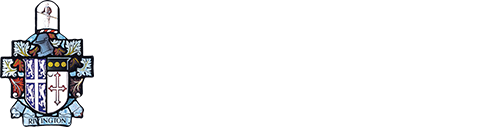Useful Links## Mathematics

Year 10 Maths Work

Year 11 Maths Work

Key Stage 5 Maths Work

Contact: Head of Mathematics - Mr J Fiddler

Subject Overview:

Mathematics is a universal language that has been developed over centuries and provides solutions to some of history’s most intriguing problems. It is the foundation of understanding the world, the ability to reason mathematically, an appreciation of the beauty and power of mathematics, and a sense of enjoyment and curiosity about the subject.

The core aim of the curriculum is to build fluency in the fundamental skills of mathematics. This will be through clear modelling of skills, frequent practice and introduction of complex problems. Students should be able to reason mathematically and be able to justify their solutions by following a line of enquiry and using proof. Students should then build up a full understanding of how they can solve problems by applying their mathematics to a variety of routine and non-routine problems of increasing difficulty by breaking down problems in smaller understandable steps.

Students are encouraged to make plenty of contributions in lessons, to listen carefully to the ideas of others, and to critically reflect upon contributions. We explicitly teach learners the appropriate ways to talk like mathematicians, and to listen to one another's ideas and reasoning.

### Key Stage 3 Overview:

We follow the National Curriculum for Mathematics at Key Stage 3.

Students build on their KS2 study, and are taught to:

• Develop fluency
• Reason mathematically
• Solve problems

We teach the following topics:

• Number
• Statistics
• Probability
• Geometry and measures
• Ratio, proportion and rates of change
• Algebra

### Key Stage 4 Overview:

We follow the Edexcel specification for Mathematics. All students are entered for either the Foundation tier or the Higher tier GCSE at the end of Year 11.

The Edexcel GCSE encourages learners to develop confidence in, and a positive attitude towards, Mathematics and to recognise the importance of mathematics in their own lives and to society. It also provides a strong mathematical foundation for learners who go on to study Mathematics at a higher level post-16. This specification enables learners to:

• develop fluent knowledge, skills and understanding of mathematical methods and concepts
• acquire, select and apply mathematical techniques to solve problems
• reason mathematically, make deductions and inferences and draw conclusions
• comprehend, interpret and communicate mathematical information in a variety of forms appropriate to the information and context.